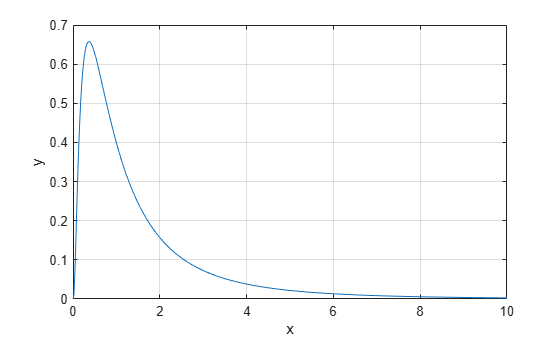# lognpdf

Lognormal probability density function

## Syntax

``y = lognpdf(x)``
``y = lognpdf(x,mu)``
``y = lognpdf(x,mu,sigma)``

## Description

````y = lognpdf(x)` returns the probability density function (pdf) of the standard lognormal distribution, evaluated at the values in `x`. In the standard lognormal distribution, the mean and standard deviation of logarithmic values are 0 and 1, respectively.```
````y = lognpdf(x,mu)` returns the pdf of the lognormal distribution with the distribution parameters `mu` (mean of logarithmic values) and 1 (standard deviation of logarithmic values), evaluated at the values in `x`.```

example

````y = lognpdf(x,mu,sigma)` returns the pdf of the lognormal distribution with the distribution parameters `mu` (mean of logarithmic values) and `sigma` (standard deviation of logarithmic values), evaluated at the values in `x`.```

## Examples

collapse all

Compute the pdf values evaluated at the values in `x` for the lognormal distribution with mean `mu` and standard deviation `sigma`.

```x = 0:0.02:10; mu = 0; sigma = 1; y = lognpdf(x,mu,sigma);```

Plot the pdf.

```plot(x,y) grid on xlabel('x') ylabel('y')```## Input Arguments

collapse all

Values at which to evaluate the pdf, specified as a positive scalar value or an array of positive scalar values.

To evaluate the pdf at multiple values, specify `x` using an array. To evaluate the pdfs of multiple distributions, specify `mu` and `sigma` using arrays. If one or more of the input arguments `x`, `mu`, and `sigma` are arrays, then the array sizes must be the same. In this case, `lognpdf` expands each scalar input into a constant array of the same size as the array inputs. Each element in `y` is the pdf value of the distribution specified by the corresponding elements in `mu` and `sigma`, evaluated at the corresponding element in `x`.

Example: `[-1,0,3,4]`

Data Types: `single` | `double`

Mean of logarithmic values for the lognormal distribution, specified as a scalar value or an array of scalar values.

To evaluate the pdf at multiple values, specify `x` using an array. To evaluate the pdfs of multiple distributions, specify `mu` and `sigma` using arrays. If one or more of the input arguments `x`, `mu`, and `sigma` are arrays, then the array sizes must be the same. In this case, `lognpdf` expands each scalar input into a constant array of the same size as the array inputs. Each element in `y` is the pdf value of the distribution specified by the corresponding elements in `mu` and `sigma`, evaluated at the corresponding element in `x`.

Example: `[0 1 2; 0 1 2]`

Data Types: `single` | `double`

Standard deviation of logarithmic values for the lognormal distribution, specified as a positive scalar value or an array of positive scalar values.

To evaluate the pdf at multiple values, specify `x` using an array. To evaluate the pdfs of multiple distributions, specify `mu` and `sigma` using arrays. If one or more of the input arguments `x`, `mu`, and `sigma` are arrays, then the array sizes must be the same. In this case, `lognpdf` expands each scalar input into a constant array of the same size as the array inputs. Each element in `y` is the pdf value of the distribution specified by the corresponding elements in `mu` and `sigma`, evaluated at the corresponding element in `x`.

Example: `[1 1 1; 2 2 2]`

Data Types: `single` | `double`

## Output Arguments

collapse all

pdf values, evaluated at the values in `x`, returned as a scalar value or an array of scalar values. `y` is the same size as `x`, `mu`, and `sigma` after any necessary scalar expansion. Each element in `y` is the pdf value of the distribution specified by the corresponding elements in `mu` and `sigma`, evaluated at the corresponding element in `x`.

collapse all

### Lognormal Distribution

The lognormal distribution is a probability distribution whose logarithm has a normal distribution.

The probability density function (pdf) of the lognormal distribution is

`$y=f\left(x|\mu ,\sigma \right)=\frac{1}{x\sigma \sqrt{2\pi }}\mathrm{exp}\left\{\frac{-{\left(\mathrm{log}x-\mu \right)}^{2}}{2{\sigma }^{2}}\right\},\text{ }\text{for}\text{\hspace{0.17em}}x>0.$`

## Alternative Functionality

• `lognpdf` is a function specific to lognormal distribution. Statistics and Machine Learning Toolbox™ also offers the generic function `pdf`, which supports various probability distributions. To use `pdf`, create a `LognormalDistribution` probability distribution object and pass the object as an input argument or specify the probability distribution name and its parameters. Note that the distribution-specific function `lognpdf` is faster than the generic function `pdf`.

• Use the Probability Distribution Function app to create an interactive plot of the cumulative distribution function (cdf) or probability density function (pdf) for a probability distribution.

 Mood, A. M., F. A. Graybill, and D. C. Boes. Introduction to the Theory of Statistics. 3rd ed., New York: McGraw-Hill, 1974. pp. 540–541.

 Evans, M., N. Hastings, and B. Peacock. Statistical Distributions. 2nd ed., Hoboken, NJ: John Wiley & Sons, Inc., 1993.